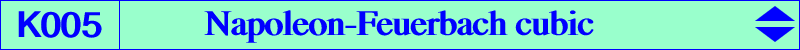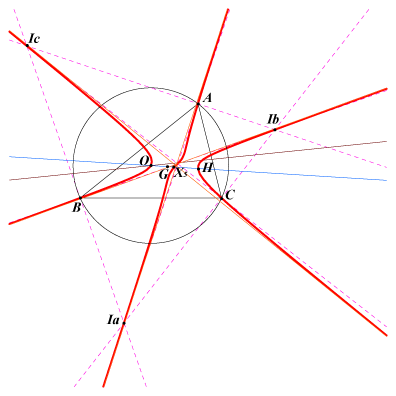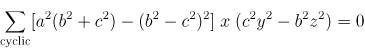See the related K397 for another equation See also K584 for a trilinear equation and related commentsX(1), X(3), X(4), X(5), X(17), X(18), X(54), X(61), X(62), X(195), X(627), X(628), X(2120), X(2121), X(3336), X(3459), X(3460), X(3461), X(3462), X(3463), X(3467), X(3468), X(3469), X(3470), X(3471), X(3489), X(3490), X(6191), X(6192), X(7344), X(7345), X(8837), X(8839), X(8918), X(8919), X(8929), X(8930), X(38931), X(38932), X(38933), X(38934), X(38935), X(39261), X(39262), X(46035), X(46036), X(46037), X(47304), X(47305), X(47306), X(47307), see table below Ia, Ib, Ic excenters four foci of the MacBeath inconic : X(3), X(4) and two imaginary Ha, Hb, Hc projections of G on the altitudes and their isogonal conjugates Oa, Ob, Oc. These points are the vertices of the orthocentroidal and orthocentroidal-isogonic triangles. They also lie on K397. centers of the 6 equilateral triangles erected on the sides of ABC, externally (Ae, Be, Ce) or internally (Ai, Bi, Ci), see figure below centers of equilateral cevian and precevian (anticevian) triangles (Jean-Pierre Ehrmann). See table 10, table 14. Ix-anticevian points and their isogonal conjugates : see table 23 X(5)-circumcevian points (see Q141) ***The Napoleon-Feuerbach cubic is the isogonal pK with pivot X(5) = nine-point center. See Table 27. It is anharmonically equivalent to the Neuberg cubic, see Table 20. Its isotomic transform is pK(X76, X311) and its Psi transform is Q041. The symbolic substitution SS{a -> √a} transforms K005 into K1081.Locus properties : (see also Z. Cerin's paper in the bibliography) Denote by PaPbPc the pedal triangle of point P. The Euler lines of APbPc, BPcPa, CPaPb concur (at Q) if and only if P lies on the Napoleon cubic (together with the line at infinity). H, P, Q are collinear and the locus of Q is Q093. Compare with K001, property 5. Under the homothety h(P,-2), PaPbPc is transformed into QaQbQc which is perspective to ABC if and only if P lies on the Napoleon cubic. For this reason, the Napoleon cubic is called -2-pedal cubic in Pinkernell's paper. The perspector lies on K060 which is the 2-cevian cubic. Pa, Pb, Pc are the reflections of point P in the sidelines of triangle ABC. Oa, Ob, Oc are the circumcenters of triangles PaBC, PbCA, PcAB. The triangles ABC and OaObOc are perspective if and only if P lies on K060 (together with the circumcircle and the line at infinity). The locus of the perspector is the Napoleon cubic. The cevian lines of point P meet the perpendicular bisectors of ABC at A', B', C'. The locus of P such that the sum of oriented lines angles (BA',BC)+(CB',CA)+(AC',AB) = pi/2 (mod. pi) is the Napoleon cubic. When this sum is 0 (mod. pi), we obtain the Brocard (second) cubic K018. (Jean-Pierre Ehrmann) Let Q be a fixed point, QaQbQc its pedal triangle and P a variable point. The parallel to QbQc through P intersects AB and AC at Ab and Ac. A1 (resp. A2) is the apex of the equilateral triangle erected outwardly (resp. inwardly) on AbAc. Similarly define B1, B2 and C1, C2. The two triangles ABC, A1B1C1 (resp. A2B2C2) are perspective for all P if and only if Q lies on the Napoleon cubic. The perspector lies on K129b (resp. K129a). (Problem raised by Antreas Hatzipolakis, Hyacinthos #10560) An orthopivotal cubic O(P) is a pK if and only if its orthopivot P lies on the Napoleon cubic. The singular focus of O(P) lies on Q041, the Psi-transform of K005. Let P be a variable point. Reflect B in PC, C in PB and denote by La the perpendicular bisector of these two points. Lb, Lc are defined cyclically. La, Lb, Lc concur (at Q) if and only if P lies on K005, together with the circumcircle and the line at infinity. (Ngo Quang Duong, ADGEOM #3309). The locus of Q is Q114, together with the Euler line and the Jerabek hyperbola. K005 is the Laplacian of the quintic Q003 i.e. the locus of points whose polar conic in the quintic is a rectangular hyperbola. Let P be a point. Denote by Bc and Cb the reflections of B in the line PC and C in the line PB respectively. (Ca) is the circumcircle of triangle ABcCb and (Cb), (Cc) are defined likewise. These three circles are congruent if and only if P lies on K005 (Kadir Altintas, 2021-01-12). Let PaPbPc be the anticevian triangle of a point P and denote by Qa, Qb, Qc the reflections of Pa, Pb, Pc in the sidelines of ABC respectively. ABC and QaQbQc are perspective (at Q) if and only if P lies on K005. The locus of Q is K060. (Angel Montesdeoca, 2021-08-02). Note that PaPbPc and QaQbQc are perspective (at Q') if and only if P lies on K002. The locus of Q' is K004. Let PaPbPc be the pedal triangle of a point P. Denote by Xa the point X(74) of triangle APbPc and define Xb, Xc cyclically. P, Xa, Xb, Xc lie on a same circle if and only if P lies on K005 (Kadir Altintas, 2021-07-24).Points on the Napoleon cubic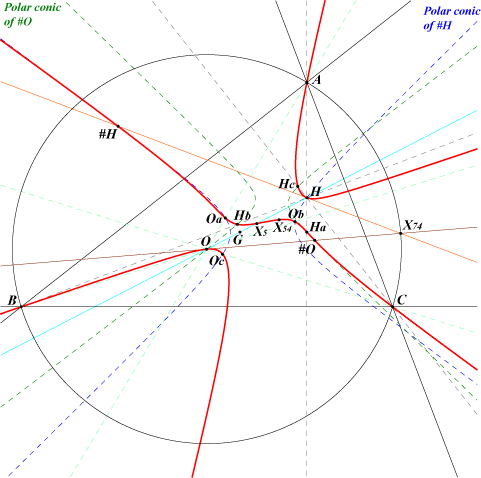Altitudes and cevians of O K005 contains : – Ha, Hb, Hc projections of G on the altitudes of ABC – #O = X(3470), the common tangential of O and Ha, Hb, Hc – Oa, Ob, Oc isogonal conjugates of Ha, Hb, Hc, these points on the cevians of O – #H = X(38933), the common tangential of H and Oa, Ob, Oc Note that the tangents at O and H pass through X(74) and that #O, #H and X(54) are collinear (they are the tangentials of O, H and X(5)) on the satellite line of the Euler line. – (#O)* = X(3471) and (#H)*, isogonal conjugates of #O and #H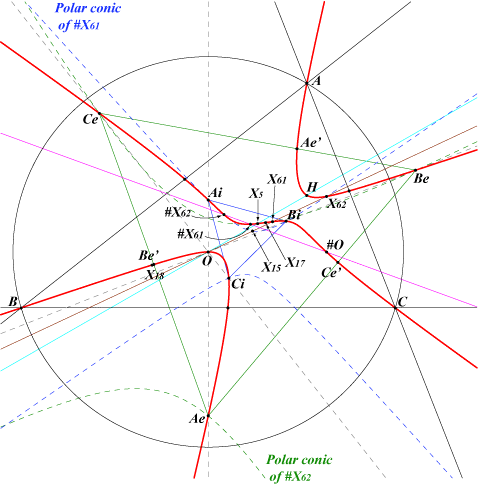Napoleon triangles K005 contains : – the vertices of the outer triangle AeBeCe. The tangents at Ae, Be, Ce, X61 concur at #X61 on the curve. – the vertices of the inner triangle AiBiCi. The tangents at Ai, Bi, Ci, X62 concur at #X62 on the curve. – the third points Ae', Be', Ce' on the sidelines of AeBeCe (these two triangles are perspective at X61). – the third points Ai', Bi', Ci' on the sidelines of AiBiCi (these two triangles are perspective at X62). Note that #O is also the perspector of the triangles Ae'Be'Ce' and Ai'Bi'Ci' and that #O, #X61, #X62 are collinear on the satellite line of the Brocard line.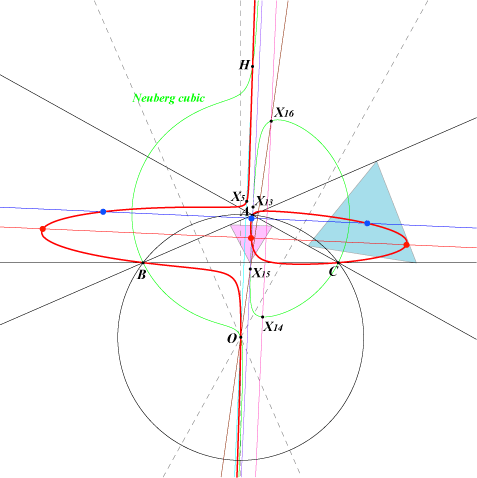Centers of the equilateral cevian or anticevian triangles K005 contains the six centers (not always real as seen in the figure) of the equilateral cevian points i.e. the points (such as X(370)) whose cevian triangle is equilateral. These equilateral cevian points lie on the Neuberg cubic. These centers lie on the perpendicular bisectors of X(13)X(15) (red points) and X(14)X(16) (blue points). See Table 10 for more details.   K005 also contains the six centers (not always real) of the equilateral anticevian points i.e. the points whose anticevian triangle is equilateral. See Table 14 for more details and a figure.   This gives 24 points on K005, counting the isogonal conjugates.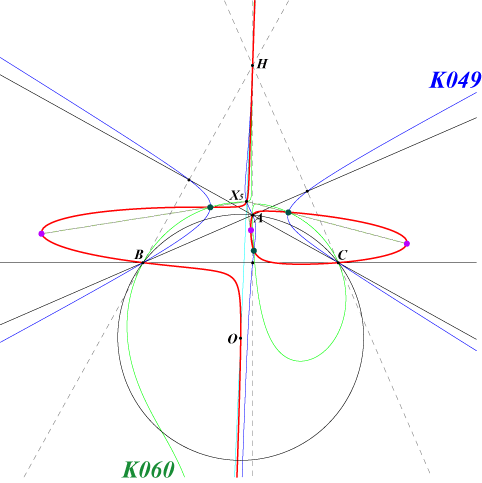Ix-anticevian points K005 contains the four (green points, not always real) Ix-anticevian points. These points are the common points (apart A, B, C, H, X5) of the cubics of the pencil which contains K005, K049, K060 and many other cubics. See Table 23 for explanations. Obviously K005 also contains the isogonal conjugates (purple points) of these four points. This gives eight more points on the cubic.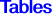The table gives centers on the cubic. Each weak point (in red) must have its three extraversions on the curve. The SEARCH value is given for these points. Most of the points are the perspectors of two inscribed triangles in the cubic. The A-vertex is given in the table. P* is the isogonal conjugate of P and P/Q is the cevian quotient of P and Q.number 0 1 2 3 4 5 6 7 8 9 10 11 12 triangle A Ia A-cevian of X(5) Ha Oa Ae Ai Ae* Ai* Ae' Ai' Ae'* Ai'*triangles SEARCH value center on K005 comments 1 8 -70.58390235756 X5/X6192 on X1-X18 -43.97562105826 X3467 on X1-X195 -35.17663270180 X3471 on X4-X195, isogonal of the tangential of O 0 9 -30.55984867257 X38931 on X3-X628 2 5 -25.54564173887 X8839 = X5/X61 on X4-X18 and X54-X61 4 8 7 11 2 3 -19.15788099913 X195 X5/X3 7 8 4 11 -17.90134341250 0 6 -16.28067399778 X18 -11.96959001257 X7345 1 11 -10.89453772135 -9.21852338354 on X1-X628 -8.75340796080 (X5/X18)* -6.18775905247 X54 2 4 -6.03883592346 X3462 = X5/X4 on X4-X54 and X195-X2121 0 3 -5.67661941093 X4 6 9 -5.65363167559 5 11 -5.64425182639 3 8 -5.29953617839 X8930 on X3-X18 7 10 8 11 -4.58243757196 on X61-X3490 -3.90988152470 (X5/X3489)* -3.70747719830 X5/X7344 -2.90770500510 (X5/X6191)* -2.34250300590 (X5/X7344)* 1 10 -2.33728658165 -2.21235552520 X5/X3489 -2.08848250120 (X5/X3467)* 6 12 -1.95442776483 -1.64508530484 X7344 =X6192* -1.34076932689 X3463 = X3462* on X3-X2120 -0.78316288550 on X1-X627 -0.62737826095 X3489 = X627* on X5-X627 -0.60900246355 X47304 = (#H)* isogonal of the tangential of H 8 10 -0.50252073688 5 10 -0.38889052256 0 8 -0.37470151131 X62 3 5 6 10 -0.30754962330 X3459 = X195* on X4-X2120 and X5-X195 -0.24819038319 X5/X3463 1 3 -0.13439182998 X3336 on X1-X3 -0.09644156557 (X5/X6192)* -0.01673252978 X5/X3490 3 7 -0.00396742485 X8929 on X3-X17 8 9 4 5 0.00380995175 X8919 on X4-X61 6 11 0.01423970330 X3490 = X628* on X5-X628 2 6 0.03185131329 X8837 = X5/X62 on X4-X17 and X54-X62 4 7 8 12 0.04216393294 X5/X3459 1 4 0.05065713778 X3468 = X5/X3336 on X1-X4 7 12 0.34430234915 1 7 0.49615812266 X5/X6191 on X1-X17 2 9 0.49936417563 #X17 tangential of X17 11 12 0.50243416641 #H = X38933 tangential of H 0 2 0.55287174163 X5 3 4 5 7 6 8 9 11 10 12 1 5 0.68838123821 X6191 on X1-X61 2 12 0.69363920522 10 11 0.78847648057 0.86588575053 X2121 X2120* 0.90047708562 (X5/X61)* 0 11 0.94522557017 #X61 = X39262 tangential of X61 9 10 0.97735900985 X3470 tangential of O 1.12427146000 (X5/X7345)* 1 12 1.29989476029 2 10 1.31531857256 #X18 tangential of X18 1.32753457700 X3461 = (X5/X1)* 0 5 1.38665455007 X17 0 1 1.69030850946 X1 4 6 1.81224721118 X8918 on X4-X62 5 12 7 9 1.84130663013 1.87961569030 X2120 X5/X54 2 11 1.89737799592 0 7 1.92170828086 X61 3 6 5 9 1 9 1.99862864623 2.00016007300 X5/X7345 2.09841058440 (X5/X17)* 0 10 2.35123193553 X38932 9 12 3.10001130310 3 12 5.11479727733 X627 4 9 6 7 1 6 5.27357128758 X6192 on X1-X62 5.44356595290 X5/X3467 5.58159639350 (X5/X3463)* 0 4 6.78236289420 X3 5 6 3 9 7.08392671609 4 12 7.34387740579 2 8 7.63837849959 X5/X18 on X18-X54 3 10 8.53849280298 9.36263622100 (X5/X62)* 10.37403549600 (X5/X3459)* 0 12 11.80585277728 #X62 = X39261 tangential of X62 2 7 14.69984666597 X5/X17 on X17-X54 16.26406858488 X3469 = X3468* 3 11 40.44945437302 X628 4 10 5 8 98.67000462400 (X5/X3490)* 1 2 113.96312522690 X3460 = X5/X1 on X1-X54